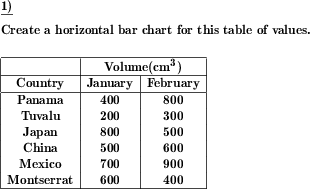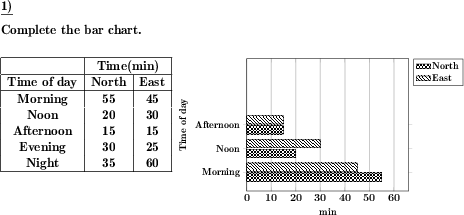Custom math worksheets at your fingertips# Details for problem "Bar chart for multi data set table of values"

Quickname: 2404

Elementary School, Primary School, Junior High School, Middle School, High School.

## Summary

Create a bar chart for a given table of values with multiple data sets.

## Examples## Description

Comparison of data is an important concept and to compare discrete data, bar charts are used. A table of values is given that contains multiple sets of data and assigns discrete terms such as cities, months, or weekdays to variable units of measurement such as length, weight, or time.

The task is to create a suitable bar chart that shows the discrete terms of the table on the Y-axis and the variable values of the different data sets on the X-axis.

This problem type provides variation in the level of problems and hence can be used for differentiation abilities in grades 5 to 7. Small groups are a good option to serve these worksheets as well as independent learners. Practicing these worksheets will build up the following skills.

1. Make a bar chart by using provided table values.
2. Extend the bar chart by observing the existing part of the bar chart.
3. Recognize a bar graph from other graphs and charts.
4. Interpretation of bar graph with its components.

If desired, parts of the diagram can be provided with the problem statement. So it is possible

• to provide no diagram
• to provide a diagram without the bars, where only the labeling of the X or Y axes or both are specified
• to provide a diagram with axis labels where one of the bars is already drawn in

As additional help, a note can be included in the task definition on how to label the axes. The size of the bar chart can be selected in three steps. The answer key will include the bar chart based on given table values.

Download free printable worksheets for this math problem here. The worksheet contains the problems only, the solution sheet includes the answers. Just click on the respective link.

•Worksheet 1Solution sheet with answers
•Worksheet 2Solution sheet with answers
•Worksheet 3Solution sheet with answers

If you can not see the solution sheets for download, they may be filtered out by an ad blocker that you may have installed. If this is the case, please allow ads for this page and reload the page. The solution sheets will then reappear.

• Do these sample worksheets do not really fit?
• Do you need more math worksheets, with a different level of difficulty?
• Would you like to combine different problems on a worksheet and adjust them to your needs?
• As a teacher, you can put together your own worksheets using the automatically generated math problems provided.
With a free initial credit, you can start creating your own math worksheets in a few minutes.

You can try it for free! Register here, to create custom worksheets now!

## Customization options for this problem

Parameter
Possible values
Note on axis labeling
Yes, No
Provide diagram
none, no axis labels, X axis labeled, Y axis labeled, both axes labeled, part of diagram
Size of diagram
small, medium, large
Number of data sets
2, 3, 4

## Similar problems

Remark
Description
With one data set only
Create a bar chart for a given table of values.
Reversed problem statement: Read values from diagram and create a table of values
Read values from a bar chart with multiple data sets and create or complete a table of values.
Same, but with a vertical bar chart or column chart
Create a column diagram or vertical bar chart for a given table of values with multiple data sets.